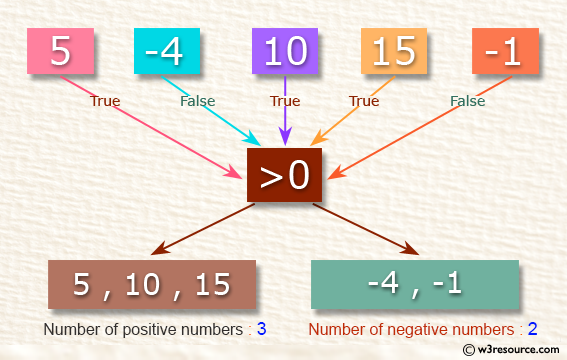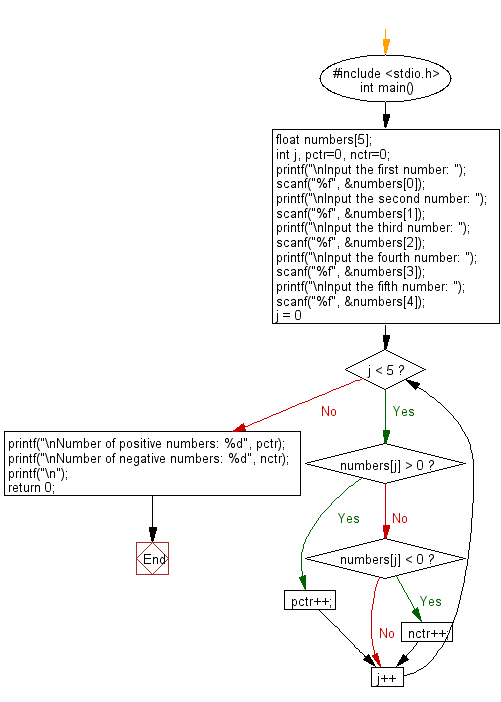﻿ C : Count the positive and negative numbers in five numbers# C Exercises: Counts the number of positive and negative numbers

## C Basic Declarations and Expressions: Exercise-27 with Solution

Write a C program that read 5 numbers and counts the number of positive numbers and negative numbers.

Pictorial Presetation:C Code:

``````#include <stdio.h>
int main() {
float numbers;
int j, pctr=0, nctr=0;
printf("\nInput the first number: ");
scanf("%f", &numbers);
printf("\nInput the second number: ");
scanf("%f", &numbers);
printf("\nInput the third number: ");
scanf("%f", &numbers);
printf("\nInput the fourth number: ");
scanf("%f", &numbers);
printf("\nInput the fifth number: ");
scanf("%f", &numbers);
for(j = 0; j < 5; j++) {
if(numbers[j] > 0)
{

pctr++;
}
else if(numbers[j] < 0)
{
nctr++;
}
}
printf("\nNumber of positive numbers: %d", pctr);
printf("\nNumber of negative numbers: %d", nctr);
printf("\n");
return 0;
}
``````

Sample Output:

```Input the first number: 5

Input the second number: -4

Input the third number: 10

Input the fourth number: 15

Input the fifth number: -1

Number of positive numbers: 3
Number of negative numbers: 2
```

Flowchart:C Programming Code Editor:

What is the difficulty level of this exercise?

Test your Programming skills with w3resource's quiz.

﻿

## C Programming: Tips of the Day

How to initialize array to 0 in C?

Global variables and static variables are automatically initialized to zero. If you have simply-

`char ZEROARRAY;`

at global scope it will be all zeros at runtime. But actually there is a shorthand syntax if you had a local array. If an array is partially initialized, elements that are not initialized receive the value 0 of the appropriate type. You could write:

`char ZEROARRAY = {0};`

The compiler would fill the unwritten entries with zeros. Alternatively you could use memset to initialize the array at program startup:

Ref : https://bit.ly/3cvqplP